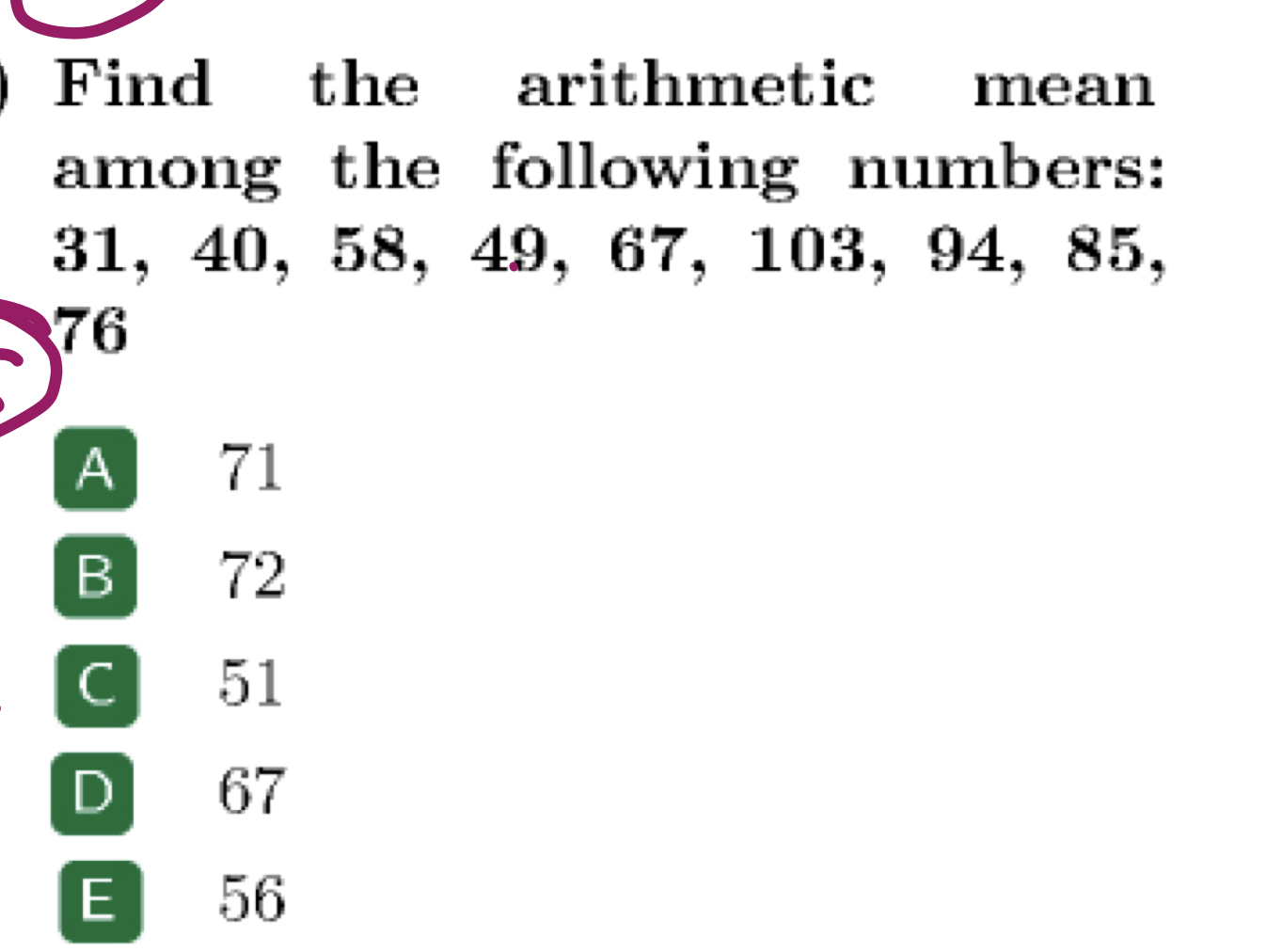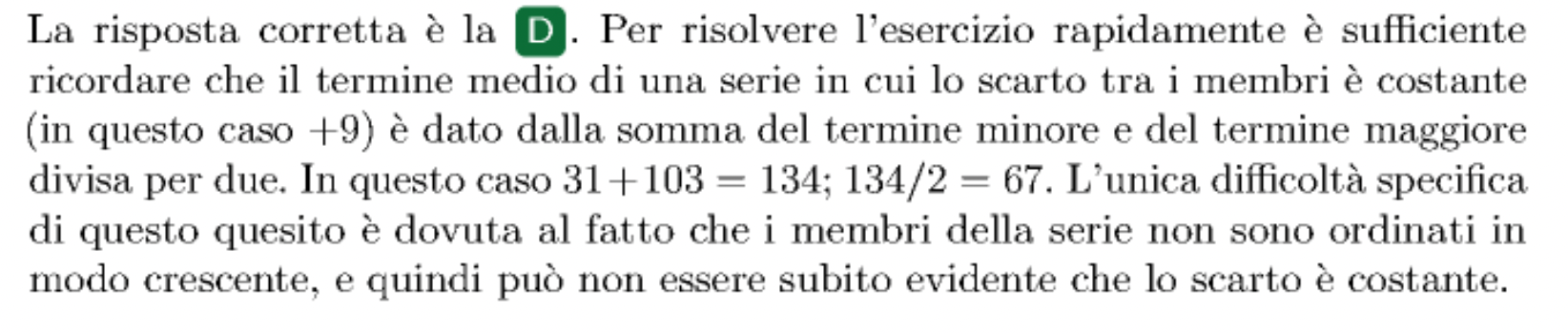# Logic/ math questionthe answer is D and the explanation is given in Italian below it. what is the logic behind it, I solved it the usual math way but the explanation shows smth else

1 Like

Hey!
Here we should find The number that is in the middle of all other numbers… which is 67

in this case, the question says arithmetic mean isn’t that sum of all/# of numbers pretty much the average?

Yes exactly …
This question is asking median… so in my point of view it’s wrong.

1 Like

Since the consecutive terms differ by constant quantity (+9), we just need to take the first and the last number, add them both and divide the sum of two by 2, to calculate the mean. So: 31+103=134, 134/2=67 What makes this question tricky is the order of the consecutive numbers.

1 Like

grazie per la traduzione1 Like